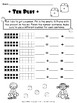# Kindergarten 10 FRAMES!Subject
Resource Type
File Type

PDF

(6 MB|3 pages)
Standards
• Product Description
• StandardsNEW

This packet contains 3 different pages and ways of adding ten using ten frames.

It is suitable to Kindergarten and First Grade.

Understand the meaning of the equal sign, and determine if equations involving addition and subtraction are true or false. For example, which of the following equations are true and which are false? 6 = 6, 7 = 8 - 1, 5 + 2 = 2 + 5, 4 + 1 = 5 + 2.
The numbers from 11 to 19 are composed of a ten and one, two, three, four, five, six, seven, eight, or nine ones.
Compose and decompose numbers from 11 to 19 into ten ones and some further ones, e.g., by using objects or drawings, and record each composition or decomposition by a drawing or equation (e.g., 18 = 10 + 8); understand that these numbers are composed of ten ones and one, two, three, four, five, six, seven, eight, or nine ones.
Count to answer “how many?” questions about as many as 20 things arranged in a line, a rectangular array, or a circle, or as many as 10 things in a scattered configuration; given a number from 1-20, count out that many objects.
Total Pages
3 pages
N/A
Teaching Duration
N/A
Report this Resource to TpT
Reported resources will be reviewed by our team. Report this resource to let us know if this resource violates TpT’s content guidelines.